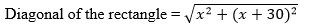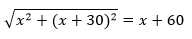Guru

# The diagonal of a rectangular field is 60 metres more than the shorter side. If the longer side is 30 metres more than the shorter side, find the sides of the field. Q.6

• 0

How i solve this question of class 10th of quadratic equation. It is very important type of question. The diagonal of a rectangular field is 60 metres more than the shorter side. If the longer side is 30 metres more than the shorter side, find the sides of the field.

Share

1. Let us say, the shorter side of the rectangle be x m.

Then, larger side of the rectangle = (x + 30) mAs given, the length of the diagonal is = x + 30 m

Therefore,⇒ x2 + (x + 30)2 = (x + 60)2

⇒ x2 + x2 + 900 + 60x = x2 + 3600 + 120x

⇒ x2 – 60x – 2700 = 0

⇒ x2 – 90x + 30x – 2700 = 0

⇒ x(– 90) + 30(x -90) = 0

⇒ (– 90)(x + 30) = 0

⇒ = 90, -30

However, side of the field cannot be negative. Therefore, the length of the shorter side will be 90 m.

and the length of the larger side will be (90 + 30) m = 120 m.

• 0